Jekyll2023-01-15T10:45:07-08:00https://wendy-xiao.github.io/feed.xmlWen Xiao’s Personal WebsiteA personal website for Wen XiaoWen Xiaowendyxiao0609@gmail.comChu-Liu/Edmonds’ Algorithm for Max Spanning Tree in Di-graph2020-07-10T00:00:00-07:002020-07-10T00:00:00-07:00https://wendy-xiao.github.io/posts/chuliuemdond_algorithmIn this blog, I will introduce the algorithm to find the maximum spanning tree in the directed graphs - the Chu-Liu/Edmonds’ Algorithm.

### Problem Definition

Given a connected directed graph $G=\{V,E\}$ with vertices $V=\{v_1,v_2,...,v_n\}$ and edges $E=\{e_{12},...,e_{ij}\}$, in which $e_{ij}$ represents the edge from vertex $v_i$ to $v_j$ with weight $w(e_{ij})$ the goal is to find the maximum spanning tree $T=\{V,E^t\}$ where all the vertices are connected, each node (except the root) has only one incoming edge, and the sum of weights of the edges is the maximum.

### A Step-by-Step Explanation of Chu-Liu/Edmonds’ Algorithm

The Chu-Liu/Edmonds’ algorithm is designed in a recursive manner, to better explain the idea, I’ll show the algorithm step-by-step with an example.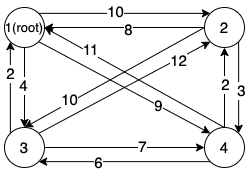An example of a fully connected directed graph with four vertices.

#### Step Zero

Given the graph shown above, the first step is to decide the start point, i.e. the root of the tree. It can be predefined by the user, otherwise, the root is the node with the highest sum of outgoing edges, $r=\arg\max_{v_i\in V}\sum_{v_j \in V} e_{ji}$. Then as the root can only have the outgoing edges, we remove all the incoming edges of the root.

In this case, the root is node 1 and after removing the unnecessary edges, the resulting graph is shown as: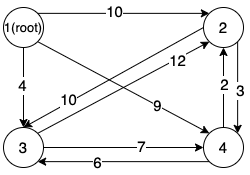#### Step One

First, we start from the graph $MG$ with the maximum incoming edge for each node (other than the root), i.e. for each node $v_i$, there is only one incoming edge from node $\pi(v_i)$, denoting $e_{\pi(v_i)v_i}$, and it is the edge with the maximum weight. If the graph is a tree, then it is the maximum spanning tree, otherwise, the graph contains at least one circle, then we need to break the circles by replacing certain edges with edges outside the graph $MG$.

Back to the example, the green edges in the following graph forms the graph $MG$, and we can find a circle between node $2$ and node $3$.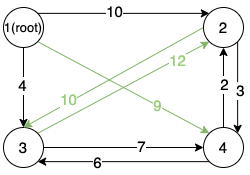#### Step Two (recursive call)

Randomly picking one circle $C_{node}=\{v_{c_1},v_{c_2},...v_{c_k}\}$ in $MG$, the circle itself is optimal, and we only need to break the circle with the minimum cost. To achieve this, we first build a new graph $G'$ by treating the circle as a new node $v_C$, and then find the maximum spanning tree $A$ in the new graph $G'$ (recursively run the algorithm on $G'$).

Now we first decribe the way to build new graph $G'=\{V',E'\}$ with the vertex set $V'=V \setminus C\cup\{v_C\}$. As for the edges $E'$, we split them into three cases:

1. For edge $e_{sd}$ in $E$, if $s\notin C_{node}$ and $d\in C_{node}$, then we add an edge $e_{sv_C}'$ to $E'$ with weight $w(e_{sv_C}')=w(e_{sd})-w(e_{\pi(v_d)v_d})$ (it is a negative value)
2. For edge $e_{sd}$ in $E$, if $s\in C_{node}$ and $d\notin C_{node}$, then we add an edge $e_{v_Cd}'$ to $E'$ with weight $w(e_{v_C d}')=w(e_{sd})$
3. For edge $e_{sd}$ in $E$, if $s\notin C_{node}$ and $d\notin C_{node}$, then we add an edge $e_{sd}'$ to $E'$ with weight $w(e_{sd}')=w(e_{sd})$

Then there might be multiple edges between $v_C$ and other nodes, we only keep the edge with maximum weight between $v_C$ and each other node.

In this example, there is only one circle formed by node $2$ and node $3$, so we treat the circle as a new node $v_C$, as shown in the left figure below. Then by applying the rules mentioned above, we build the new graph $G'$ shown in the left. Apparently there are multiple edges between node $1,4$ and node $v_C$, then we only keep the one with the highest weight (i.e. the blue edges, and the number in the parenthesis represents the original source/destinition of the edge in the circle).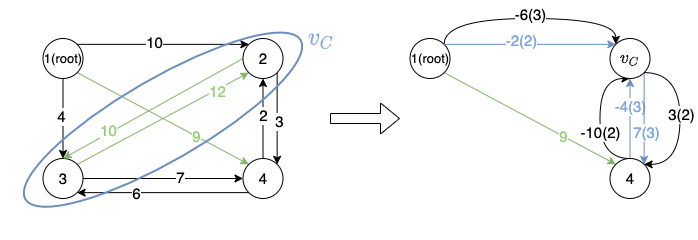Then we need to find the maximum spanning tree $A$ for the new graph $G'$, and it is formed by the edges in red in the figure below.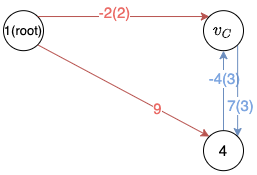#### Step Three

Without loss of generality, assume the incoming edge of $v_C$ in $A$ is from node $v_s$ and its corresponding edge in the original graph $G$ is $e_{sk}$, with $v_k \in C_{node}$, then the edges of the final tree is formed by the combination of edges in $A$ (replacing the edges from/to $v_C$ to the original edge) and the edges in the circle without the incoming edge of node $v_k$.

As shown in the graph below, $E^t$ is formed by the red edges in the right figure.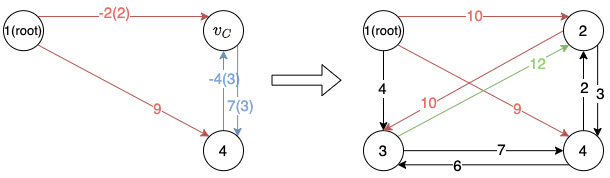We got it! Congrats! ^.^

### Python Implementation

In this section, I’ll show my python implementation of the algorithm. There must be more efficient implementations, and discussions on improving the implementation are welcome.

### Summary

In this blog, I introduced the traditional algorithm for finding the maximum/minimum spanning tree in the directed graph, and showed a python implementation of the algorithm. The algorithm is often used in the NLP area, especially in the topic like syntactic parsing/discourse parsing. Discussions on the implementation, the algorithm, or the applications are welcome.

### Reference

 https://en.wikipedia.org/wiki/Edmonds%27_algorithm Wikipedia of Edmonds’ algorithm

]]>
Wen Xiaowendyxiao0609@gmail.com